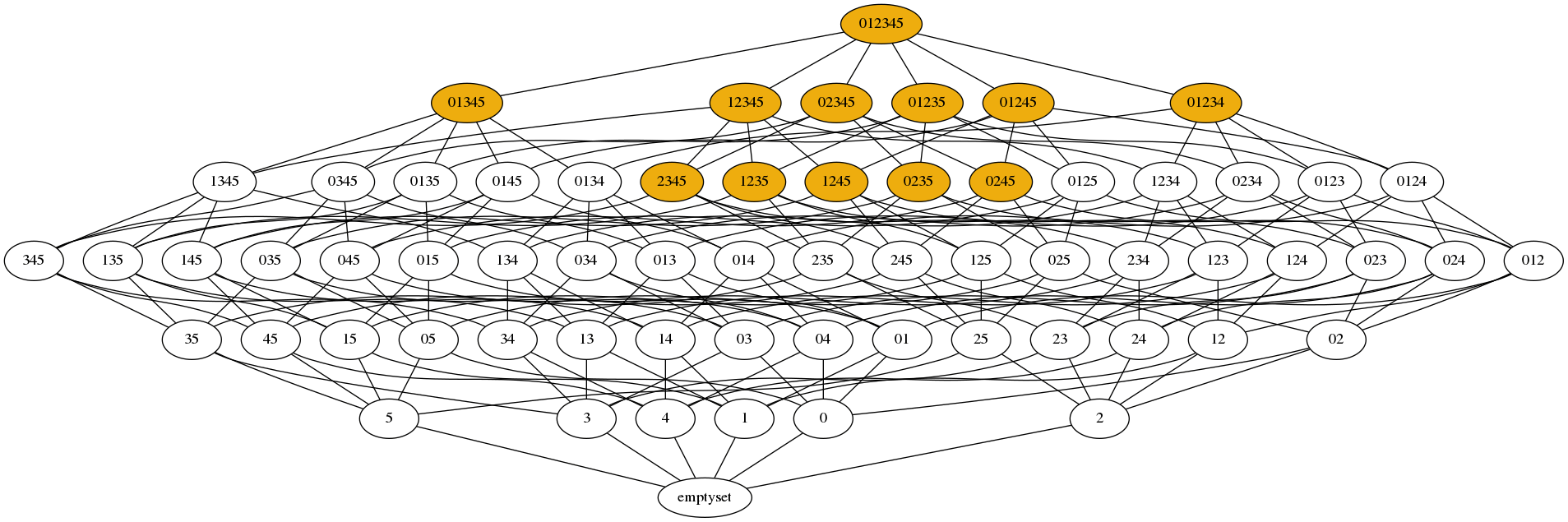# #StackBounty: #boolean-functions #matching #monotone Perfect matching of monotone Boolean function with null Euler characteristic

### Bounty: 100

For a set $$V = {0,ldots,k}$$ of variables, let $$mathbf{G}_V$$ be the undirected graph with set of vertices $${S subseteq V}$$ and set of edges $${{S,S’} mid S subseteq S’ text{ and }|S’| = |S|+1}$$, in other words the underlying undirected graph of the Hasse diagram of the powerset of $$V$$. Let $$phi$$ be a Boolean function on variables $$V$$, that we see as a set of subsets of $$V$$. Define the graph $$mathbf{G}_V[phi]$$ to be the subgraph of $$mathbf{G}_V$$ induced by $$phi$$.

I conjecture the following:

If $$phi$$ is monotone and $$sum_{S in phi} (-1)^{|S|} = 0$$, i.e., $$phi$$ has as many satisfying valuations of even and odd size, then $$mathbf{G}_V[phi]$$ or $$mathbf{G}_V[lnotphi]$$ has a perfect matching.

What I know:

• For a Boolean function $$phi$$, $$sum_{S in phi} (-1)^{|S|}$$ is called the Euler characteristic of $$phi$$.
• Observe that $$sum_{S in phi} (-1)^{|S|} = – sum_{S in lnotphi} (-1)^{|S|}$$ ($$=0$$ in our case).
• Of course, if $$sum_{S in phi} (-1)^{|S|} neq 0$$, then neither $$mathbf{G}_V[phi]$$ nor $$mathbf{G}_V[lnotphi]$$ can have a perfect matching.
• My claim does not hold if we do not impose $$phi$$ to be monotone. For example, consider the following Boolean function (the satisfying valuations of $$phi$$ are colored):$$sum_{S in phi} (-1)^{|S|} = 0$$, but $$mathbf{G}_V[phi]$$ does not have a perfect matching since, for instance, $${3,4}$$ is isolated. The same holds for $$mathbf{G}_V[lnotphi]$$ by considering $${0,3,4}$$.

• There are examples where both have a perfect matching, and examples where only one does. Here is one such example (this is actually the smallest such example):$$sum_{S in phi} (-1)^{|S|} = 0$$, $$mathbf{G}_V[phi]$$ does not have a perfect matching, but $$mathbf{G}_V[lnotphi]$$ does (trust me).
I suspect that, if the claim is true, what decides if $$mathbf{G}_V[phi]$$ or $$mathbf{G}_V[lnotphi]$$ has a perfect matching is $$max(|phi|,|lnotphi|)$$.

• There are no counterexamples to my claim for $$k leq 5$$.

• In the particuliar case where $$lnot phi$$ corresponds to a collapsible abstract simplicial complex (an abstract simplicial complex is just another word for the negation of a monotone Boolean function), then $$mathbf{G}_V[lnotphi]$$ has a perfect matching.

What I have tried:

Since $$mathbf{G}_V[phi]$$ and $$mathbf{G}_V[lnotphi]$$ are bipartite, I’ve tried using:

• Kőnig’s theorem. So here I would need to show that for $$mathbf{G}_V[phi]$$ (or $$mathbf{G}_V[lnotphi]$$), there is no vertex cover of size less that $$|phi|/2$$ (since the set of all subsets in $$phi$$ of even size is obviously a vertex cover of $$mathbf{G}_V[phi]$$).
• Hall’s mariage theorem. Here I would need to show that, for every subset $$B$$ of $$phi$$ containing only subsets of even size, the subsets of $$B$$ are (collectively) adgacent to at least $$|B|$$ vertices in $$mathbf{G}_V[phi]$$.

with no luck so far. I should add that I don’t have any particular reason for why this should be true, but this came to me as an intriguing question while I was working on something else.

Get this bounty!!!

This site uses Akismet to reduce spam. Learn how your comment data is processed.